# User:IssaRice/Computability and logic/Semantic completeness

Semantic completeness is sometimes written as: if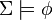$\Sigma \models \phi$, then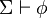$\Sigma \vdash \phi$.

Semantic completeness is the completeness that is the topic of Godel's completeness theorem.

Semantic completeness differs from negation completeness.

Semantic completeness is about the completeness of a logic (not about the completeness of a theory).

## Definition

I want to make sure all these definitions are saying the same thing, so let me list some from several textbooks so I can explicitly compare.

Smith's definition: a logic is semantically complete iff for any set of wffs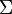$\Sigma$ and any sentence$\phi$, if$\Sigma \models \phi$ then$\Sigma\vdash\phi$.

Leary/Kristiansen's definition: A deductive system consisting of logical axioms$\Lambda$ and a collection of rules of inference is said to be complete iff for every set of nonlogical axioms$\Sigma$ and every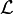$\mathcal L$-formula$\phi$, if$\Sigma \models \phi$, then$\Sigma \vdash \phi$.

## Alternative formulation

It is possible to formulate completeness by saying that a consistent set of sentences is satisfiable. In other words, the following are equivalent:

1. Let$\Gamma$ be a set of sentences, and let$\phi$ be a sentence. If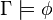$\Gamma \models \phi$, then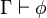$\Gamma \vdash \phi$.
2. Let$\Gamma$ be a set of sentences. If$\Gamma$ is consistent, then$\Gamma$ is satisfiable (has a model).

### Proof idea

The first trick in the proof is to notice that the$\Gamma$ that appears in (1) is not the same as the$\Gamma$ that appears in (2). Since$\Gamma$ is an arbitrary set of sentences in each of (1) and (2) (i.e. each statement is surrounded by "for all$\Gamma$ …"), we can use a different set of sentences than the arbitrary$\Gamma$ we are given. If you try to use the same$\Gamma$ in both places, your proof will go nowhere.

In other words, the statement of the proposition obfuscates the situation a bit by using the same symbol for "different things" in the proof. One might ask why one would obfuscate things this way; one response is that if you're just stating one of the formulations in isolation, you would just use your "default variable" for a set of sentences. For instance, if you tend to use$\Gamma$ for a set of sentences, then if someone asked you to state (1), you would use$\Gamma$. Then if someone asked you to state (2) a few weeks later, you wouldn't use$\Delta$ or$\Sigma$ unless you had (1) in mind.

The other trick is to figure out just what to use as$\Gamma$ when going from (2) to (1), and to figure out what to do with$\phi$ when going from (1) to (2).

The rest of the proof is some routine manipulations.

### Proof

Suppose (1) is true. We will prove the contrapositive of (2). Let$\Gamma$ be a set of sentences, and suppose$\Gamma$ is unsatisfiable. Let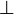$\bot$ be a contradictory sentence, such as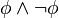$\phi\wedge\neg\phi$. Now, since$\Gamma$ is unsatisfiable, there is no structure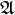$\mathfrak A$ such that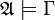$\mathfrak A \models \Gamma$. This means that vacuously, for every$\mathfrak A$ such that$\mathfrak A \models \Gamma$, we have$\mathfrak A \models \bot$. Thus we have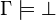$\Gamma \models \bot$. Now by (1) (with$\bot$ serving the role of "$\phi$"), this means$\Gamma \vdash \bot$, which shows that$\Gamma$ is inconsistent, as desired.

Now suppose (2) is true. Let$\Gamma$ be a set of sentences, let$\phi$ be a sentence, and suppose$\Gamma \models \phi$. We will first show that$\Gamma \cup \{\neg\phi\}$ is unsatisfiable. Suppose for the sake of contradiction that$\Gamma \cup \{\neg\phi\}$ is satisfiable. Then we would have a structure$\mathfrak A$ such that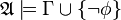$\mathfrak A \models \Gamma \cup \{\neg\phi\}$. This means$\mathfrak A \models \Gamma$ and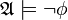$\mathfrak A \models \neg\phi$. Since$\Gamma \models \phi$, this means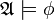$\mathfrak A \models \phi$. So we have both$\mathfrak A \models \phi$ and$\mathfrak A \models \neg\phi$, a contradiction. This proves that$\Gamma \cup \{\neg\phi\}$ is unsatisfiable.

Since$\Gamma \cup \{\neg\phi\}$ is unsatisfiable, by (2) (with$\Gamma \cup \{\neg\phi\}$ in place of "$\Gamma$") we have that$\Gamma \cup \{\neg\phi\}$ is inconsistent. This means$\Gamma \cup \{\neg\phi\} \vdash \bot$, so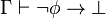$\Gamma \vdash \neg\phi\to\bot$ by the deduction theorem, which means$\Gamma \vdash \phi$, which is what we wanted. Note that the exact details of the derivations in this paragraph will differ based on the logical system one is using. For instance, going from "$\Gamma \vdash \neg\phi\to\bot$" to "$\Gamma \vdash \phi$" is not automatic; one must provide an entirely syntactic derivation in the logical system one is using.

### Proof commentary

In proving (1) ⇒ (2), we only needed that "if$\Gamma$ is unsatisfiable, then$\Gamma\models\bot$". However, it is also possible to prove the converse, and show that if$\Gamma$ is satisfiable, then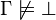$\Gamma \not\models\bot$. Some books factor out both sides of this implication as a lemma.

Similarly, in proving (2) ⇒ (1), we only needed that "if$\Gamma \models \phi$, then$\Gamma \cup \{\neg\phi\}$ is unsatisfiable", but the converse holds as well.